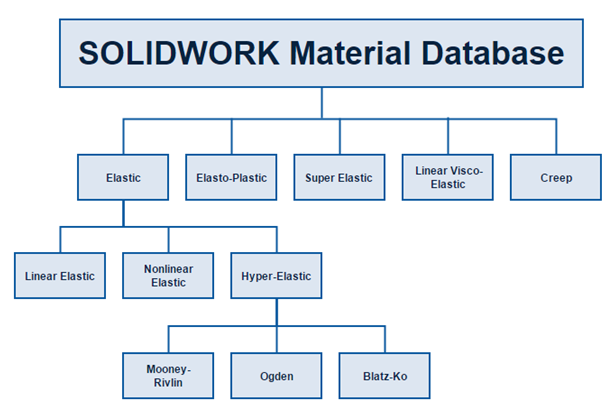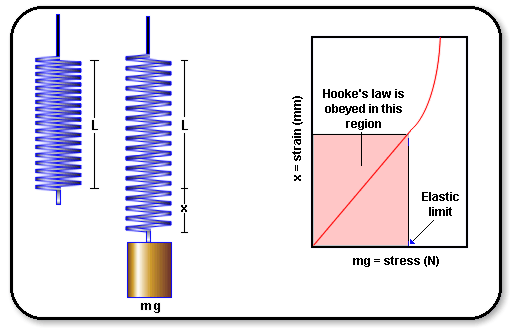When it comes to Nonlinear analysis, the #1 criteria that really drives the entire analysis is the material (properties). In this blog series, we will take a closer look at all the different material model types that can be found in the SOLIDWORKS Simulation Nonlinear module. To start, there are 5 main types of material models:

• Elastic Model
• Elasto-Plastic Model
• Super Elastic Nitinol Model
• Linear Visco-Elastic Model
• Creep Model

The different model types can be found within the SOLIDWORKS Nonlinear Simulation Material database, through the Material Properties.Within the Elastic Model material model type, there are 3 subcategories that users can choose from: Linear, Nonlinear and Hyper-Elastic. The chart below gives a representation of this material model breakdown.We will focus on the most general material model type, Linear Elastic, which can also be found in the Linear Static Module.  This material model type obeys Hooke’s law, where by stress is directly proportional to strain, as external loading is increased or decreased.This material model is suitable when no stress-strain graph input values are available, as only critical values of the material property are needed for SOLIDWORKS Simulation. However, because of this, models that experience large strain might yield incorrect results, due to the linearization of the critical values that are inputted. The Linear Elastic model is also appropriate for parts where the geometry will return to its original shape if the load is removed. Note: In my next blog, I will discuss the stress-strain graph in more detail, as we will talk about the Nonlinear Elastic material model.

For the Linear Elastic material model type to work correctly in the simulation, 4 main properties must be included:

• Elastic Modulus
• Poisson’s Ratio
• Mass Density
• Yield Strength

In addition to the required properties listed above, here are some of the key features of this material model type:

• Material behavior can be isotropic or orthotropic.
• Isotropic materials have the comparable properties in all direction of the part and deforms based on the Young’s Modulus and Poisson’s ratio.
• Orthotropic material models react independently in each direction (X, Y & Z). This material model requires the input of 9 independent material constants to ensure proper deformation of the part.
• When thermal loads are included (i.e. temperature, heat flux, heat power etc.), the thermal conductivity, coefficient of thermal expansion (CTE), and specific heat of the material has to be inputted.
• Different temperatures can be inputted to create dependence to any mechanical properties (i.e. density, Young’s modulus, shear modulus etc.). This relationship is called cross-property correlations.

With this information, you can successfully run the correct simulation on your models, and yield more accurate calculations and draw better conclusions for your designs.